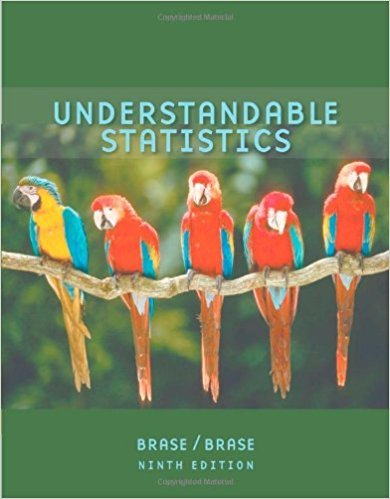×
×

# Solutions for Chapter 12.2: NONPARAMETRIC STATISTICS## Full solutions for Understandable Statistics | 9th Edition

ISBN: 9780618949922Solutions for Chapter 12.2: NONPARAMETRIC STATISTICS

Solutions for Chapter 12.2
4 5 0 276 Reviews
16
2
##### ISBN: 9780618949922

Since 11 problems in chapter 12.2: NONPARAMETRIC STATISTICS have been answered, more than 33833 students have viewed full step-by-step solutions from this chapter. Chapter 12.2: NONPARAMETRIC STATISTICS includes 11 full step-by-step solutions. This expansive textbook survival guide covers the following chapters and their solutions. Understandable Statistics was written by and is associated to the ISBN: 9780618949922. This textbook survival guide was created for the textbook: Understandable Statistics, edition: 9.

Key Statistics Terms and definitions covered in this textbook
• a-error (or a-risk)

In hypothesis testing, an error incurred by failing to reject a null hypothesis when it is actually false (also called a type II error).

A variation of the R 2 statistic that compensates for the number of parameters in a regression model. Essentially, the adjustment is a penalty for increasing the number of parameters in the model. Alias. In a fractional factorial experiment when certain factor effects cannot be estimated uniquely, they are said to be aliased.

• Average run length, or ARL

The average number of samples taken in a process monitoring or inspection scheme until the scheme signals that the process is operating at a level different from the level in which it began.

• Biased estimator

Unbiased estimator.

• Binomial random variable

A discrete random variable that equals the number of successes in a ixed number of Bernoulli trials.

• Chi-square test

Any test of signiicance based on the chi-square distribution. The most common chi-square tests are (1) testing hypotheses about the variance or standard deviation of a normal distribution and (2) testing goodness of it of a theoretical distribution to sample data

• Conditional probability distribution

The distribution of a random variable given that the random experiment produces an outcome in an event. The given event might specify values for one or more other random variables

• Conditional variance.

The variance of the conditional probability distribution of a random variable.

• Contingency table.

A tabular arrangement expressing the assignment of members of a data set according to two or more categories or classiication criteria

• Continuous distribution

A probability distribution for a continuous random variable.

• Correlation coeficient

A dimensionless measure of the linear association between two variables, usually lying in the interval from ?1 to +1, with zero indicating the absence of correlation (but not necessarily the independence of the two variables).

• Counting techniques

Formulas used to determine the number of elements in sample spaces and events.

• Covariance

A measure of association between two random variables obtained as the expected value of the product of the two random variables around their means; that is, Cov(X Y, ) [( )( )] =? ? E X Y ? ? X Y .

• Crossed factors

Another name for factors that are arranged in a factorial experiment.

• Designed experiment

An experiment in which the tests are planned in advance and the plans usually incorporate statistical models. See Experiment

• Discrete uniform random variable

A discrete random variable with a inite range and constant probability mass function.

• Error sum of squares

In analysis of variance, this is the portion of total variability that is due to the random component in the data. It is usually based on replication of observations at certain treatment combinations in the experiment. It is sometimes called the residual sum of squares, although this is really a better term to use only when the sum of squares is based on the remnants of a model-itting process and not on replication.

• Error variance

The variance of an error term or component in a model.

• Experiment

A series of tests in which changes are made to the system under study

• First-order model

A model that contains only irstorder terms. For example, the irst-order response surface model in two variables is y xx = + ?? ? ? 0 11 2 2 + + . A irst-order model is also called a main effects model

×